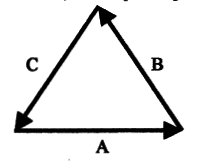# Doubling vector sums

## Homework Statement

The diagram shows 3 vector all of equal length. Which statement is true?
a. A+B=A-C
b. A+B=B-C
c. A-B=2A-C
d. A-B=2A+C
e. 2A+2B=2CNone

## The Attempt at a Solution

I just added them in my head, and thought that e. 2A+2B=2C would also work. Why doesn't it?

#### Attachments

TSny
Homework Helper
Gold Member
To see where you might have gone wrong, try the following. On a sheet of paper, draw just the two sides of the triangle representing A and B making sure you include the arrow heads. Now, on that picture, draw in the vector that represents the result of adding A and B making sure you include the arrow head on the result. Now compare your resultant vector with the vector C in the original picture.

I got the same picture?

TSny
Homework Helper
Gold Member
The resultant points in the direction that C is pointing in the image.

SammyS
Staff Emeritus
Homework Helper
Gold Member
The resultant points in the direction that C is pointing in the image.
Not correct.

TSny
Homework Helper
Gold Member
The resultant points in the direction that C is pointing in the image.

No, this is the reason you're having trouble with the problem. See the attached figure and try to answer the question shown there. If you need to, refer back to the link I gave earlier or check your notes or textbook.

#### Attachments

Oh I see, C should be pointing from the tail of A to the head of B.
But then the magnitude of C would still be the same though, so how does that help?

SammyS
Staff Emeritus
Homework Helper
Gold Member
Oh I see, C should be pointing from the tail of A to the head of B.
But then the magnitude of C would still be the same though, so how does that help?
Those equations in the Original Post are vector equations.

It's not that C should be in the opposite direction, it's that the resultant of adding A and B is -C .

haruspex
Homework Helper
Gold Member
C should be pointing from the tail of A to the head of B.
The resultant of A and B points that way. Saying C points that way when C is already defined the other way in the problem statement is confusing.
the magnitude of C would still be the same though,